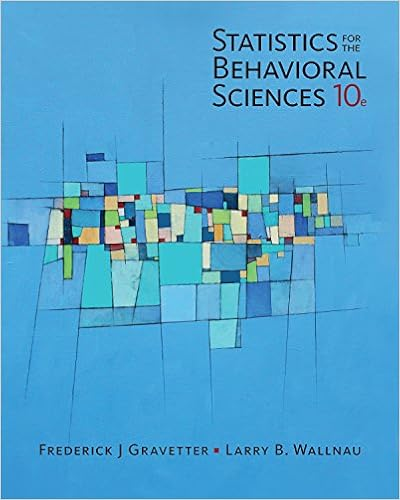# Age 15 16 17 number of students 2 5 13 7 3 x x 1 2 13

• Notes
• 166
• 100% (1) 1 out of 1 people found this document helpful

This preview shows page 70 - 76 out of 166 pages.

##### We have textbook solutions for you!
The document you are viewing contains questions related to this textbook.The document you are viewing contains questions related to this textbook.
Chapter 8 / Exercise 3
Statistics for the Behavioral Sciences
Gravetter/WallnauExpert Verified
Age (Years) 13 14 15 16 17 Number of Students 2 5 13 7 3 x x 1 2 . 13 0758 . 0 2 . 14 0704 . 0 8 . 14 0676 . 0 2 . 15 0658 . 0 1 . 16 0621 . 0 Total 3417 . 0 1 x
##### We have textbook solutions for you!
The document you are viewing contains questions related to this textbook.The document you are viewing contains questions related to this textbook.
Chapter 8 / Exercise 3
Statistics for the Behavioral Sciences
Gravetter/WallnauExpert Verified
Solution: The given distribution belongs to a grouped data and the variable involved is ages of first year students. While the number of students Represent frequencies. Ages (Years) x Number of Students f x 1 13 2 0.1538 14 5 0.3571 15 13 0.8667 16 7 0.4375 17 3 0.1765 Total 30 f 9916 . 1 1 x Now we will find the Harmonic Mean as x f f X HM = 15 0631 . 15 9916 . 1 30 Example: Calculate the harmonic mean for the given below: Marks 30-39 40-49 50-59 60-69 70-79 80-89 90-99 F 2 3 11 20 32 25 7 Solution: The necessary calculations are given below:
Marks X F x f 30-39 34.5 2 0.0580 40-49 44.5 3 0.0674 50-59 54.5 11 0.2018 60-69 64.5 20 0.3101 70-79 74.5 32 0.4295 80-89 84.5 25 0.2959 90-99 94.5 7 0.0741 Total 100 f 4368 . 1 x f Now we will find the Harmonic Mean as 60 . 69 4368 . 1 100 x f f X 4.8.6 Relationship Between The Arithmetic Mean, The Median And The Mode In a symmetrical distribution or normal distribution, that‟s peaked in the centre, the arithmetic mean = median = mode. Other features of a normal distribution are: - It is bell shaped and is divided into equal parts by the mean, mode and median 3.8.1 NORMAL DISTRIBUTION
3.8.2 POSITIVELY SKEWED DISTRIBUTION 3.8.3 NEGATIVELY SKEWED DISTRIBUTION
NB: If we have a positively skewed distribution, the arithmetic mean is not at the centre. The mean dragged to the right of centre by few high values of the variable e.g., an arithmetic mean of salaries for comparison purposes, majority (higher frequency) will be earnings less than average. The median salary would be more typical. In a negatively skewed distribution, the mean is reduced by the few small values of the variable frequency and hence will be left of the centre. The comparatively few old people features of a population in a developing country like Kenya make the mean of the population appear low, since in any case the majority in the population is young people. Again the median would be more representative. In a moderately skewed distribution the following relationship holds approximately:- (ARITHMETIC MEAN MINUS MODE = 3 (ARITHMETIC MEAN MINUS MEDIAN) OR (ARITHMETIC MEAN MODE = 3 (ARITHMETIC MEAN - MEDIAN) This formula can be used for estimating the mode from the values of the mean and the median 20 30 25 60 25 - 20 - 500 30 40 35 84 24 - 10 - 240 40 50 45 96 12 0 0 50 60 55 127 31 +10 +310 60 70 65 198 71 +20 +1420 70 - 80 75 250 52 +30 +1560 Total
4.8.7 Step-Deviation Method Here all class intervals are of the same width say 'c'. This method is employed in place of the Short-cut method. We measure all the class-marks (mid values) from some convenient value, say 'A', which generally should be taken as the class-mark of a class of maximum frequency or of a class which is the middle one. All the class marks happen to be multiples of c, since all class intervals are equal. We consider class frequencies as if they are centered at the corresponding class-marks.
•••